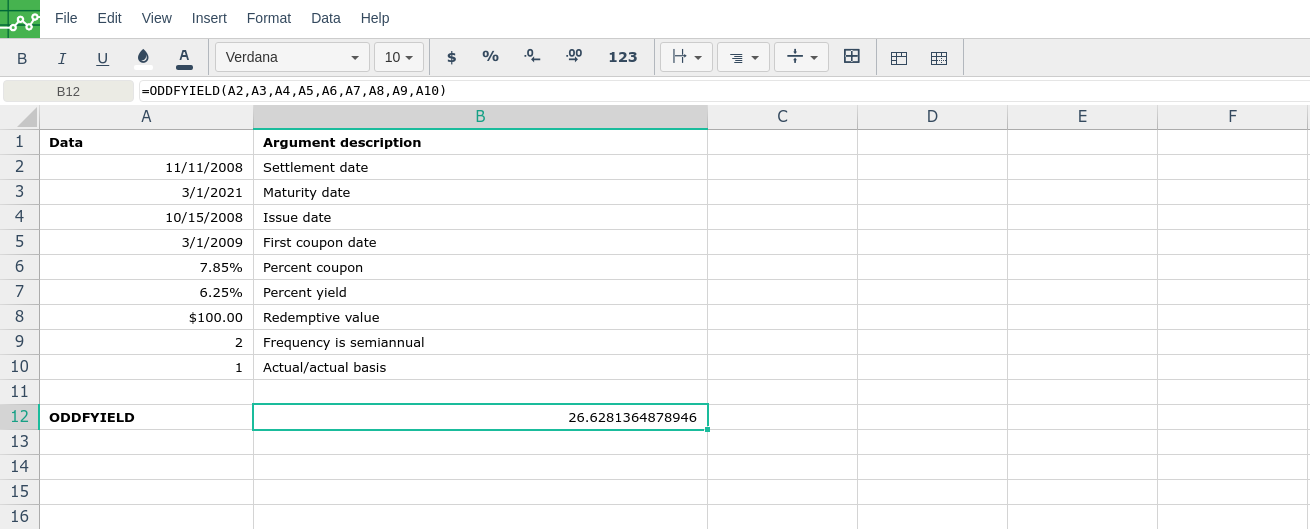# ODDFYIELD

### Description

Returns the yield of a security that has an odd (short or long) first period.

### Syntax

ODDFYIELD(settlement, maturity, issue, first_coupon, rate, pr, redemption, frequency, [basis])

IMPORTANT: Dates should be entered by using the DATE function, or as results of other formulas or functions. For example, use DATE(2008,5,23) for the 23rd day of May, 2008. Problems can occur if dates are entered as text.

The ODDFYIELD function syntax has the following arguments:

• Settlement    Required. The security's settlement date. The security settlement date is the date after the issue date when the security is traded to the buyer.

• Maturity    Required. The security's maturity date. The maturity date is the date when the security expires.

• Issue    Required. The security's issue date.

• First_coupon    Required. The security's first coupon date.

• Rate    Required. The security's interest rate.

• Pr    Required. The security's price.

• Redemption    Required. The security's redemption value per \$100 face value.

• Frequency    Required. The number of coupon payments per year. For annual payments, frequency = 1; for semiannual, frequency = 2; for quarterly, frequency = 4.

• Basis    Optional. The type of day count basis to use.

 Basis Day count basis 0 or omitted US (NASD) 30/360 1 Actual/actual 2 Actual/360 3 Actual/365 4 European 30/360

### Remarks

• Microsoft Excel stores dates as sequential serial numbers so they can be used in calculations. By default, January 1, 1900 is serial number 1, and January 1, 2008 is serial number 39448 because it is 39,448 days after January 1, 1900.

• The settlement date is the date a buyer purchases a coupon, such as a bond. The maturity date is the date when a coupon expires. For example, suppose a 30-year bond is issued on January 1, 2008, and is purchased by a buyer six months later. The issue date would be January 1, 2008, the settlement date would be July 1, 2008, and the maturity date would be January 1, 2038, which is 30 years after the January 1, 2008, issue date.

• Settlement, maturity, issue, first_coupon, and basis are truncated to integers.

• If settlement, maturity, issue, or first_coupon is not a valid date, ODDFYIELD returns the #VALUE! error value.

• If rate < 0 or if pr ≤ 0, ODDFYIELD returns the #NUM! error value.

• If basis < 0 or if basis > 4, ODDFYIELD returns the #NUM! error value.

• The following date condition must be satisfied; otherwise, ODDFYIELD returns the #NUM! error value:

maturity > first_coupon > settlement > issue

• Excel uses an iterative technique to calculate ODDFYIELD. This function uses the Newton method based on the formula used for the function ODDFPRICE. The yield is changed through 100 iterations until the estimated price with the given yield is close to the price. See ODDFPRICE for the formula that ODDFYIELD uses.

###Error.

User does not have sufficient privileges to access this Content